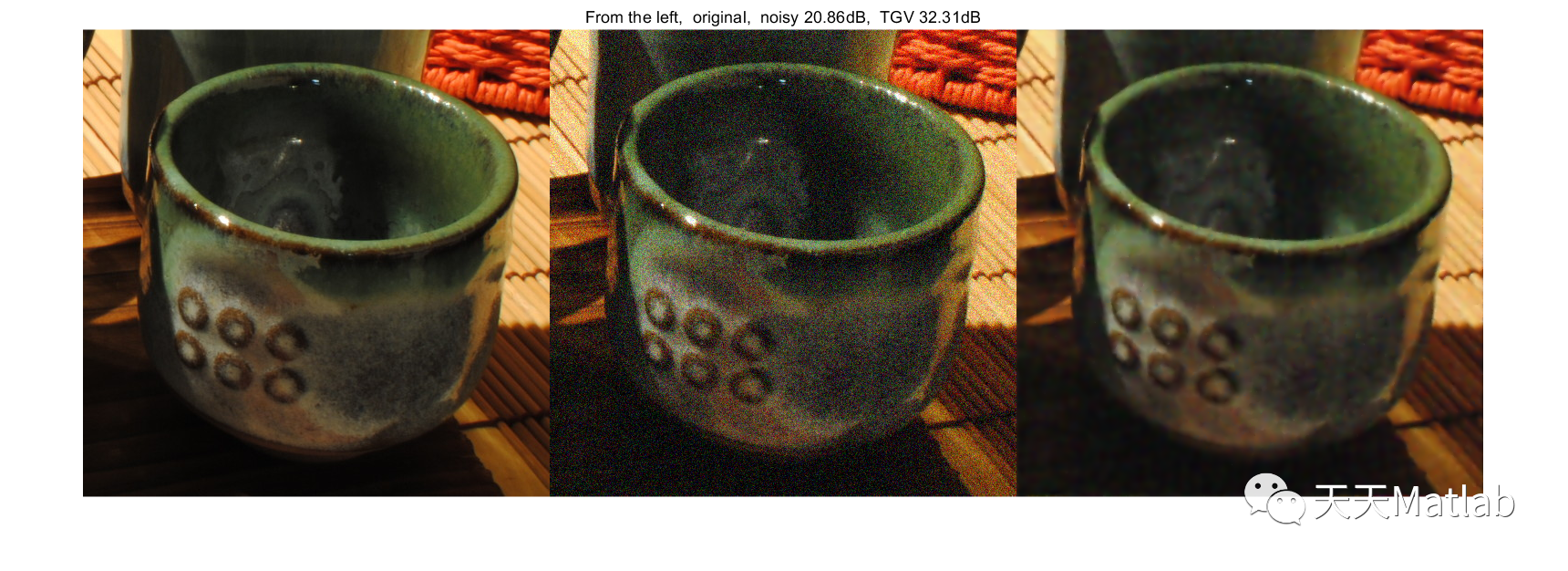# 【TGV 正则器的快速计算方法】通过FFT的总（广义）变化进行图像去噪（Matlab代码实现）

+关注继续查看

✅作者简介：热爱科研的Matlab仿真开发者，修心和技术同步精进，matlab项目合作可私信。

🍎个人主页：Matlab科研工作室

🍊个人信条：格物致知。

## 📣 部分代码

function J = rgb2ycocg( I )%RGB2YCOCG converts RGB color into YCoCg color components.% YCoCg consists of luminance Y, and chroma Co and Cg, with less correlation each other.%% J = RGB2YCOCG( I ) converts the RGB color image I into the YCoCg color image J. %ndim = ndims( I );[s1, s2, s3] = size( I );if (ndim == 3) && (s3 == 3)  sy = s1;  sx = s2;  sc = s3;  I = reshape( I, sy*sx, sc );  elseif (ndim == 2) && (s2 == 3)  sc = s2;  else  error( 'The input image have to be RGB color image' );end% t1 = [1, 1, 1] / 3;% t2 = [1, 0, -1] / 2;% t3 = [1, -2, 1] / 4;t1 = [1, 2, 1] / 4;t2 = [1, 0, -1] / 2;t3 = [-1, 2, -1] / 4;T = [t1; t2; t3];J = I * T';if (ndim == 3) && sc == 3  J = reshape( J, sy, sx, sc );endend

## ⛳️ 运行结果## 🔗 参考文献

### 👇  私信完整代码和数据获取及论文数模仿真定制

#### 9 雷达方面

##### 卡尔曼滤波跟踪、航迹关联、航迹融合|
4月前
|

【图像去噪】基于自适应小波阈值算法实现图像去噪附matlab源码
【图像去噪】基于自适应小波阈值算法实现图像去噪附matlab源码
66 0
|
4月前
|

【图像去噪】基于混合自适应（EM 自适应）实现自适应图像去噪研究（Matlab代码实现）
【图像去噪】基于混合自适应（EM 自适应）实现自适应图像去噪研究（Matlab代码实现）
27 0
|
4月前
|

【图像去噪】基于进化算法——自组织迁移算法（SOMA）的图像去噪研究（Matlab代码实现）
【图像去噪】基于进化算法——自组织迁移算法（SOMA）的图像去噪研究（Matlab代码实现）
37 0
|
5月前
|

【图像去噪】基于高斯滤波+均值滤波+中值滤波+双边滤波滤波实现图像去噪（含信噪比）附Matlab代码
【图像去噪】基于高斯滤波+均值滤波+中值滤波+双边滤波滤波实现图像去噪（含信噪比）附Matlab代码
85 0
|
6月前
|

【图像去噪】基于图像加噪去噪算法合集附matlab代码
【图像去噪】基于图像加噪去噪算法合集附matlab代码
45 0
|
7月前
|

108 0
|
7月前
|

【图像去噪】基于自适应全变分算法实现图像去噪附matlab代码
【图像去噪】基于自适应全变分算法实现图像去噪附matlab代码
58 0
|
8月前
|

【图像去噪】基于matlab 实现图像邻域滤波附matlab代码
【图像去噪】基于matlab 实现图像邻域滤波附matlab代码
66 0
|
8月前
|

【图像去噪】基于CTV-RPCA实现图像去噪和目标显著性检测附matlab代码
【图像去噪】基于CTV-RPCA实现图像去噪和目标显著性检测附matlab代码
82 0
|
10月前
|

【图像去噪】基于frost滤波器实现图像去噪附matlab代码
【图像去噪】基于frost滤波器实现图像去噪附matlab代码
101 0